# 27 Results

View
Selected filters:
• Equation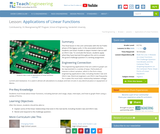Educational Use
Rating

This final lesson in the unit culminates with the Go Public phase of the legacy cycle. In the associated activities, students use linear models to depict Hooke's law as well as Ohm's law. To conclude the lesson, students apply they have learned throughout the unit to answer the grand challenge question in a writing assignment.

Subject:
Math
Material Type:
Lesson
Provider:
TeachEngineering
Provider Set:
TeachEngineering
Author:
Aubrey McKelvey
09/18/2014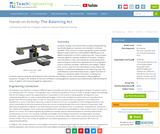Educational Use
Rating

Students visualize and interact with concepts already learned, specifically algebraic equations and solving for unknown variables. They construct a balancing seesaw system (LEGO® Balance Scale) made from LEGO MINDSTORMS® parts and digital components to mimic a balancing scale. They are given example algebraic equation problems to analyze, configure onto the balance scale, and evaluate by manipulating LEGO pieces and gram masses that represent terms of an equation such as unknown variables, coefficients and integers. Digital light sensors, built into the LEGO Balance Scale, detect any balance or imbalances displayed on the balancing scale. The LEGO Balance Scale interactively issues a digital indication of balance or imbalance within the system. If unbalanced, students continue using the LEGO Balance Scale until they are confident in their understanding of solving algebraic equations. The goal is for students to become confident in solving algebraic equations by fundamentally understanding the basics of algebra and real-world algebraic applications.

Subject:
Chemistry
Material Type:
Activity/Lab
Provider:
TeachEngineering
Provider Set:
TeachEngineering
Author:
Gisselle Cunningham
Jared Soto
Linderick Outerbridge
Russell Holstein
Timothy Li
09/18/2014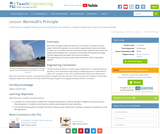Educational Use
Rating

Bernoulli's principle relates the pressure of a fluid to its elevation and its speed. Bernoulli's equation can be used to approximate these parameters in water, air or any fluid that has very low viscosity. Students learn about the relationships between the components of the Bernoulli equation through real-life engineering examples and practice problems.

Subject:
Physics
Material Type:
Lesson
Provider:
TeachEngineering
Provider Set:
TeachEngineering
Author:
Denise W. Carlson
James Prager
Karen King
09/18/2014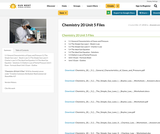Conditional Remix & Share Permitted
CC BY-NC-SA
Rating

5.1 General Characteristics of Gases and Pressure
5.2 The Simple Gas Laws I - Boyle's Law
5.3 The Simple Gas Laws II - Charles's Law
5.4 The Ideal Gas Equation
5.4 The Ideal Gas Equation - Solutions
5.5 Dalton's Law of Partial Pressure
Unit 5 Exam - Formula Sheet
Unit 5 Exam - Outline

Subject:
Chemistry
Material Type:
Activity/Lab
Unit of Study
Author:
Sun West School Division
10/17/2018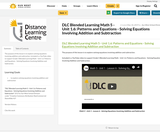Conditional Remix & Share Permitted
CC BY-NC-SA
Rating

The purpose of this lesson is to explore solving equations involving addition and subtraction.

Included is a YouTube video to support Grade 5 Blended Learning Math - Unit 1.6: Patterns and Equations - Solving Equations Involving Addition and Subtraction.

Subject:
Math
Material Type:
Activity/Lab
Homework/Assignment
Lesson
Provider:
Sun West Distance Learning Centre (DLC)
Author:
Sun West School Division
05/10/2019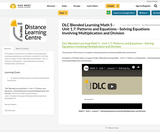Conditional Remix & Share Permitted
CC BY-NC-SA
Rating

The purpose of this lesson is to explore solving equations involving multiplication and division.

Included is a YouTube video to support Grade 5 Blended Learning Math - Unit 1.7: Patterns and Equations - Solving Equations Involving Multiplication and Division.

Subject:
Math
Material Type:
Activity/Lab
Homework/Assignment
Lesson
Provider:
Sun West Distance Learning Centre (DLC)
Author:
Sun West School Division
05/10/2019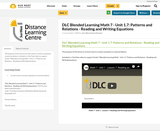Conditional Remix & Share Permitted
CC BY-NC-SA
Rating

The purpose of this lesson is to learn how to connect equations to word problems.

Included is a YouTube video to support Grade 7 Blended Learning Math - Unit 1.7: Patterns and Relations - Reading and Writing Equations.

Subject:
Math
Material Type:
Activity/Lab
Homework/Assignment
Lesson
Provider:
Sun West Distance Learning Centre (DLC)
Author:
Sun West School Division
06/07/2019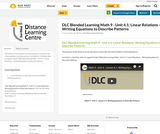Conditional Remix & Share Permitted
CC BY-NC-SA
Rating

The purpose of this lesson is to use equations to describe and solve problems involving patterns.

Included is a YouTube video to support Grade 9 Blended Learning Math - Unit 4.1: Linear Equations - Writing Equations to Describe Patterns.

Subject:
Math
Material Type:
Activity/Lab
Homework/Assignment
Lesson
Provider:
Sun West Distance Learning Centre (DLC)
Author:
Sun West School Division
06/24/2019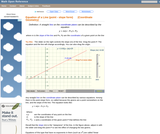Rating

An interactive applet and associated web page that demonstrate the equation of a line in point-slope form. The user can move a slider that controls the slope, and can drag the point that defines the line. The graph changes accordingly and equation for the line is continuously recalculated with every slider and / or point move. The grid, axis pointers and coordinates can be turned on and off. The equation display can be turned off to permit class exercises and then turned back on the verify the answers. The applet can be printed as it appears on the screen to make handouts. The web page has a full description of the concept of the equation of a line in point - slope form, a worked example and has links to other pages relating to coordinate geometry. Applet can be enlarged to full screen size for use with a classroom projector. This resource is a component of the Math Open Reference Interactive Geometry textbook project at http://www.mathopenref.com.

Subject:
Math
Material Type:
Simulation
Provider:
Math Open Reference
Author:
John Page
05/15/2018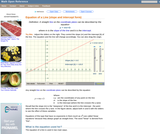Rating

An interactive applet and associated web page that demonstrate the equation of a line in coordinate geometry. The equation is in the form y=mx+b. The user can move two sliders that control a and b. The graph changes accordingly and equation for the line is continuously recalculated with every slider move. The grid, axis pointers and coordinates can be turned on and off. The equation display can be turned off to permit class exercises and then turned back on the verify the answers. The applet can be printed as it appears on the screen to make handouts. The web page has a full description of the concept of the equation of a line, a worked example and has links to other pages relating to coordinate geometry. Applet can be enlarged to full screen size for use with a classroom projector. This resource is a component of the Math Open Reference Interactive Geometry textbook project at http://www.mathopenref.com.

Subject:
Math
Material Type:
Simulation
Provider:
Math Open Reference
Author:
John Page
05/15/2018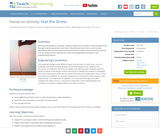Educational Use
Rating

Working individually or in groups, students explore the concept of stress (compression) through physical experience and math. They discover why it hurts more to poke themselves with mechanical pencil lead than with an eraser. Then they prove why this is so by using the basic equation for stress and applying the concepts to real engineering problems.

Subject:
Physics
Material Type:
Activity/Lab
Provider:
TeachEngineering
Provider Set:
TeachEngineering
Author:
Jeffrey Mitchell
09/18/2014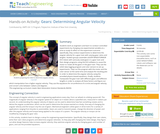Educational Use
Rating

Students work as engineers and learn to conduct controlled experiments by changing one experimental variable at a time to study its effect on the experiment outcome. Specifically, they conduct experiments to determine the angular velocity for a gear train with varying gear ratios and lengths. Student groups assemble LEGO MINDSTORMS(TM) NXT robots with variously sized gears in a gear train and then design programs using the NXT software to cause the motor to rotate all the gears in the gear train. They use the LEGO data logging program and light sensors to set up experiments. They run the program with the motor and the light sensor at the same time and analyze the resulting plot in order to determine the angular velocity using the provided physics-based equations. Finally, students manipulate the gear train with different gears and different lengths in order to analyze all these factors and figure out which manipulation has a higher angular velocity. They use the equations for circumference of a circle and angular velocity; and convert units between radians and degrees.

Subject:
Science
Material Type:
Activity/Lab
Provider:
TeachEngineering
Provider Set:
TeachEngineering
Author:
James Cox
Jasmin Mejias
Jennifer S. Haghpanah
Leonarda Huertas
Mihai Pruna
09/18/2014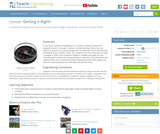Educational Use
Rating

In this lesson, students will investigate error. As shown in earlier activities from navigation lessons 1 through 3, without an understanding of how errors can affect your position, you cannot navigate well. Introducing accuracy and precision will develop these concepts further. Also, students will learn how computers can help in navigation. Often, the calculations needed to navigate accurately are time consuming and complex. By using the power of computers to do calculations and repetitive tasks, one can quickly see how changing parameters likes angles and distances and introducing errors will affect their overall result.

Subject:
Math
Material Type:
Activity/Lab
Lesson
Provider:
TeachEngineering
Provider Set:
TeachEngineering
Author:
Janet Yowell
Jeff White
Malinda Schaefer Zarske
Matt Lippis
09/18/2014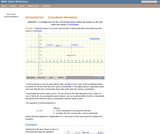Rating

An interactive applet and associated web page that show the definition of a horizontal line in coordinate geometry. The applet has two points that the user can drag which define a line. The line flagged when it is horizontal (slope=0) and the equation of the line is shown. The grid, details and coordinates can be turned on and off. The applet can be printed exactly as it appears on the screen to make handouts. The web page has a discussion on how to test for horizontal, the line equation and has links to other pages relating to coordinate geometry. Applet can be enlarged to full screen size for use with a classroom projector. This resource is a component of the Math Open Reference Interactive Geometry textbook project at http://www.mathopenref.com.

Subject:
Math
Material Type:
Simulation
Provider:
Math Open Reference
Author:
John Page
05/15/2018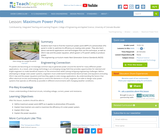Educational Use
Rating

Students learn how to find the maximum power point (MPP) of a photovoltaic (PV) panel in order to optimize its efficiency at creating solar power. They also learn about real-world applications and technologies that use this technique, as well as Ohm's law and the power equation, which govern a PV panel's ability to produce power.

Subject:
Environmental Science
Material Type:
Lesson
Provider:
TeachEngineering
Provider Set:
TeachEngineering
Author:
Abby Watrous
Jack Baum
Malinda Schaefer Zarske
Stephen Johnson
William Surles
09/18/2014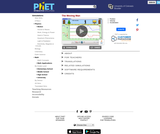Rating

Learn about position, velocity, and acceleration graphs. Move the little man back and forth with the mouse and plot his motion. Set the position, velocity, or acceleration and let the simulation move the man for you.

Subject:
Physical Science
Material Type:
Simulation
Provider:
Provider Set:
PhET Interactive Simulations
Author:
Carl Wieman
Danielle Harlow
Kathy Perkins
Sam Reid
10/04/2005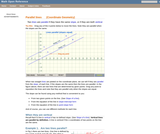Rating

An interactive applet and associated web page that demonstrate the properties of parallel lines in coordinate geometry. The applet presents two lines, each defined by a pair of draggable points. If they are parallel the slopes are the same and the applet adds the parallel arrow marks to them. The slope of each line is continuously recalculated and the case of vertical lines (with undefined slope) is handled correctly. The is a software 'detent' that snaps the lines to parallel if they are close to it. The grid, axis pointers and coordinates can be turned on and off. The slope calculation can be turned off to permit class exercises and then turned back on the verify the answers. The applet can be printed as it appears on the screen to make handouts. The web page has a full description of the method for determining if lines are parallel, a worked example and has links to other pages relating to coordinate geometry. Applet can be enlarged to full screen size for use with a classroom projector. This resource is a component of the Math Open Reference Interactive Geometry textbook project at http://www.mathopenref.com.

Subject:
Math
Material Type:
Simulation
Provider:
Math Open Reference
Author:
John Page
05/15/2018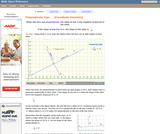Rating

An interactive applet and associated web page that show how to determine of one line is perpendicular to another in coordinate geometry. The principle used is that if two lines a re perpendicular to each other the slope of one is the negative reciprocal of the other. The applet shows to lines that the user can move. The slopes are continuously calculated as you drag them, and if the they are parallel they change color. The calculation is shown on screen updated continuously as you drag. The grid, axis pointers and coordinates can be turned on and off. The calculation display can be turned off to permit class exercises and then turned back on the verify the answers. The applet can be printed as it appears on the screen to make handouts. The web page has a full description of the concept of perpendicularity, a worked example and has links to other pages relating to coordinate geometry. Applet can be enlarged to full screen size for use with a classroom projector. This resource is a component of the Math Open Reference Interactive Geometry textbook project at http://www.mathopenref.com.

Subject:
Math
Material Type:
Simulation
Provider:
Math Open Reference
Author:
John Page
05/15/2018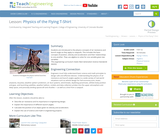Educational Use
Rating

Students are introduced to the physics concepts of air resistance and launch angle as they apply to catapults. This includes the basic concepts of position, velocity and acceleration and their relationships to one another. They use algebra to solve for one variable given two variables.

Subject:
Physics
Material Type:
Lesson
Provider:
TeachEngineering
Provider Set:
TeachEngineering
Author:
Brandi Jackson
Denise W. Carlson
Jonathan MacNeil
Scott Duckworth
Stephanie Rivale
09/18/2014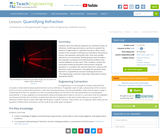Educational Use
Rating

Students learn the relevant equations for refraction (index of refraction, Snell's law) and how to use them to predict the behavior of light waves in specified scenarios. After a brief review of the concept of refraction (as learned in the previous lesson), the equations along with their units and variable definitions, are introduced. Student groups work through a few example conceptual and mathematical problems and receive feedback on their work. Then students conduct the associated activity during which they practice using the equations in a problem set, examine data from a porous film like those used in biosensors, and apply the equations they learned to a hypothetical scenario involving biosensors.

Subject:
Physics
Material Type:
Lesson
Provider:
TeachEngineering
Provider Set:
TeachEngineering
Author:
Caleb Swartz